Instantaneous Active and Reactive Power Measuring Method in Three Phase Power System

A. TAHRI, A. DRAOU

Applied Power Electronics Laboratory, Department of Electrotechnics,

University of Sciences and Technology of Oran

BP 1505 El Mnaouar (31000 Oran), ALGERIA, Fax:+213–41–421581

Contact author: Dr. Azeddine Draou, Senior member IEEE, Email: adraou@yahoo.com

Abstract

This paper describes an electronic means of measuring the instantaneous active and reactive power absorbed by any electrical equipment. The measurements are based on the Clark (a-b) and Park (d-q) transformations. The system is useful to teach electrical machines in Park’s coordinates and it allows also the study and control of some power electronics converters that are connected to three phase power network, such as static VAR compensator. The principle of the measuring method of the active and reactive power is described, and analyzed for different tests. The effectiveness of the proposed measuring method is confirmed by experimental investigation employing a test system.

Keywords

Active power, Reactive power, SVC, Clark and Park, EPROM

Introduction

Up to now, the reactive power doesn’t really have a physical significance, but it remains recognized as an essential factor in the conception and the efficient operating of AC electric network . The application of Clark (a-b) and Park (d-q) transforms to three phase system in order to calculate the instantaneous active and reactive power is a useful tool for study and analysis of many electrical systems . There are many industrial applications that require the knowledge of the instantaneous value of the active and reactive power. In fact, they are used to manage the economical aspect of their system [1, 3, 4].

The instantaneous active and reactive powers are also used in the control of converters connected to electric network . These converters can control the flow of active and reactive power in the power system to improve voltage regulation, and increasing transient stability margin [4, 5].

In this paper, an inexpensive electronic circuit that calculate Clark (a-b), Park (d-q) components and the instantaneous active and reactive power for three phase AC system is presented and discussed thoroughly.

The effectiveness of the proposed electronic method that calculates the instantaneous active and reactive currents and power is confirmed by experimental results through a laboratory prototype.

Theoretical Analysis

a-b transformation

Voltages and currents can be transformed from abc system to a-b coordinates as follows, where X denotes voltage or current:(1)

d-q transformation

From a-b transformation the d-q coordinates are given by:(2)

Active and reactive power

The active and reactive power for three phase balanced system can be written in d-q coordinates as follows, where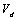,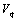,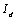and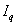are the voltages and currents in d-q coordinates: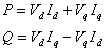(3)

Proposed Measuring System

General description

The block diagram of the proposed measuring system is presented in fig. 1. The whole system is divided in three subsystems connected in cascade.  The function of subsystem 1 is to synchronize all of the system to the AC mains using PLL and to generate the adequate addresses to generate sine and cosine functions which are stored in two EPROMS.

In the subsystem 2, the measured voltages and currents are transformed from abc to Clark and Park coordinates.  And in the third subsystem the instantaneous active and reactive power are calculated.

Detailed description

Subsystem 1:

Subsystem 1 is frequency synthesizer. The clock frequency is 16.16.16.8.50Hz = 1.638 MHz synchronized to the AC main. This clock frequency is used to drive the address generator. The address generator is composed of four 74HC161 synchronous pre-settable binary counters and two 74HC245 buffers. The addresses obtained are suitable to read in parallel two EPROMs of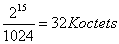, where sine and cosine functions are stored. Hence the obtained address generator is shown in fig. 2.

Subsystem 2:

The measured voltages and currents in abc frame are transformed in Clark and Park coordinates. The Clark transform given by equation (1) is obtained using simple operational amplifiers as shown in fig. 3.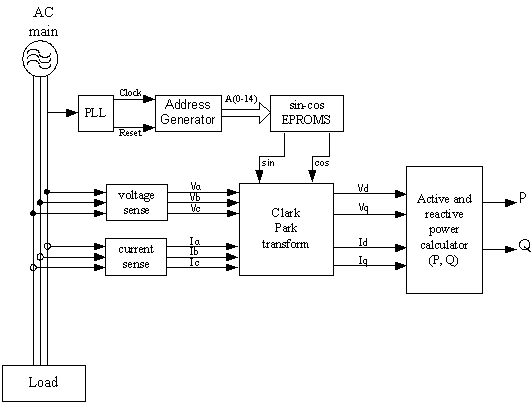Figure 1. Proposed measurement system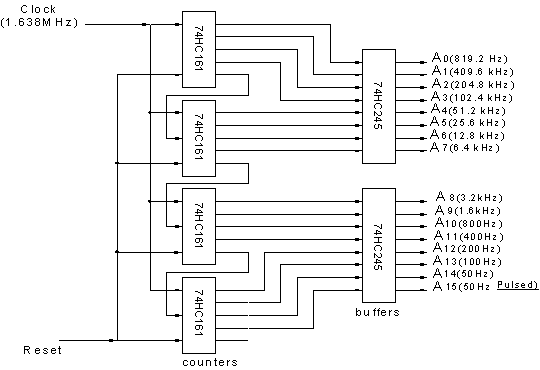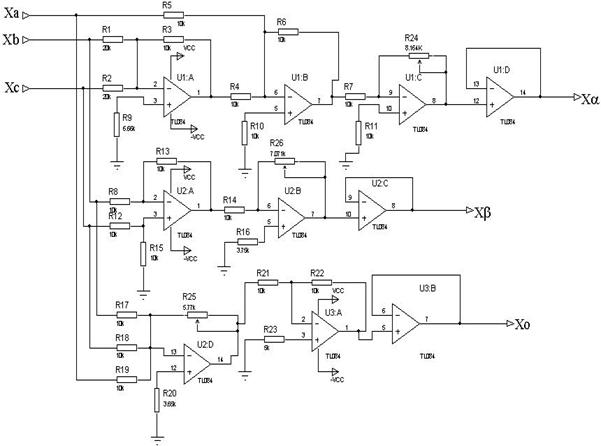Figure 3. Clark transform Circuit

Park coordinates are obtained from Clark coordinates by using equation (2).

The sine and cosine functions generated by the address generator and synchronized to the AC mains are used to obtain the Park coordinates by using the analog multiplier AD534JD as shown in fig. 4.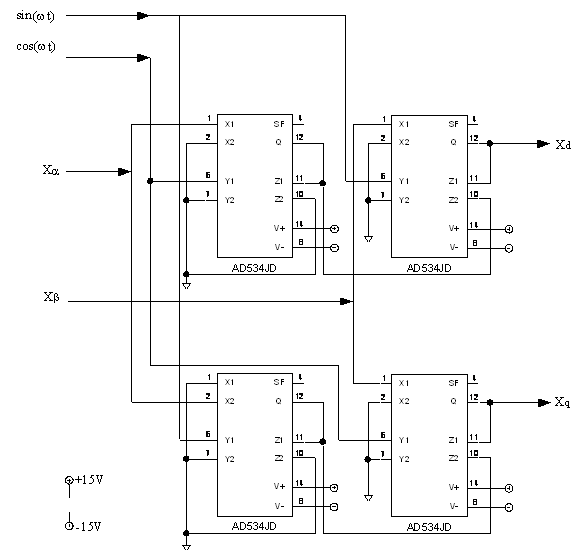Figure 4. Park transform Circuit

Subsystem 3:

The instantaneous active and reactive power calculator is also obtained using the analog multiplier AD534JD as shown in fig. 5.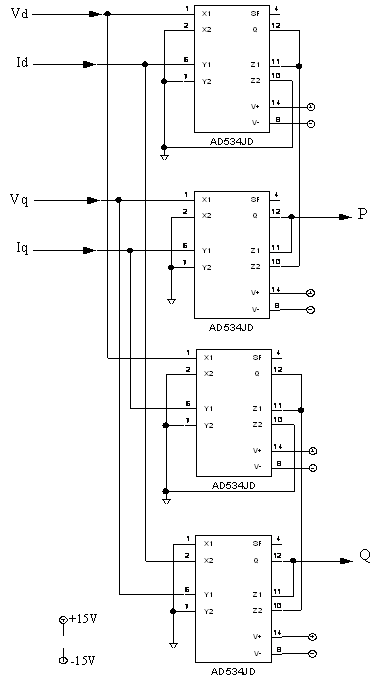Figure 5. Instantaneous active and reactive power calculator

Experimental Results

To confirm the effectiveness of the proposed measuring method of instantaneous active and reactive units, some experiments were carried out employing a test system with different loads. Fig. 6 shows the direct and quadrature Park’s voltages of the AC mains. A transient starting test of induction motor was carried at the laboratory with the proposed system.

Fig. 7 shows the AC current absorbed by induction motor in transient starting. Fig. 8 shows the direct and quadrature currents of induction motor starting.

The active and reactive power absorbed by the induction motor in transient starting are depicted in fig. 9. The measurement system was tested with induction motor without load, it is clear that the active power absorbed by the motor is negligible but there is a little amount of reactive power necessary to create the three phase  rotating magnetic field in the stator of the induction motor. Another test was carried out in the laboratory with three phase symmetric thyristor rectifier. Fig. 10 shows the output DC voltage and input current of the rectifier for the phase angle control α = 80 degree.

Fig. 11 shows the direct and quadrature currents absorbed by the three phase symmetric rectifier for the phase angle control α = 80 degree, and the active and reactive power are depicted in fig. 12.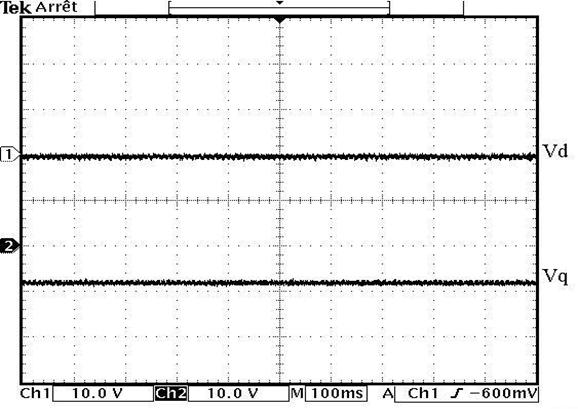Figure 6. Direct and quadrature voltages of the AC mains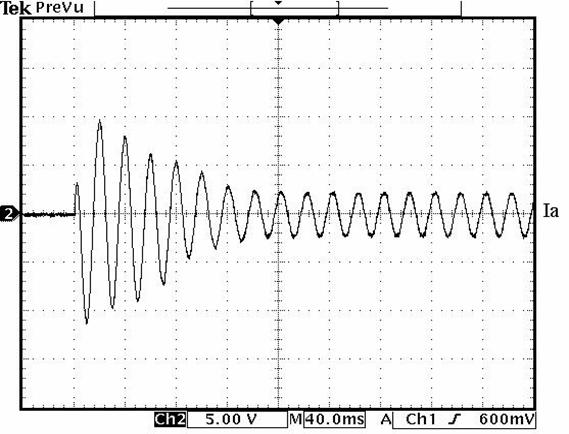Figure 7. AC current of induction motor at transient starting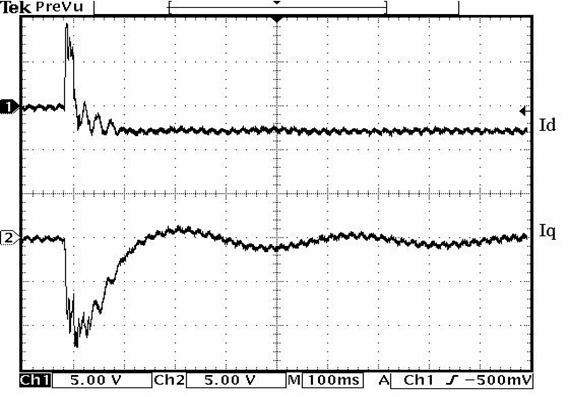Figure 8. Direct and quadrature currents of induction motor in transient starting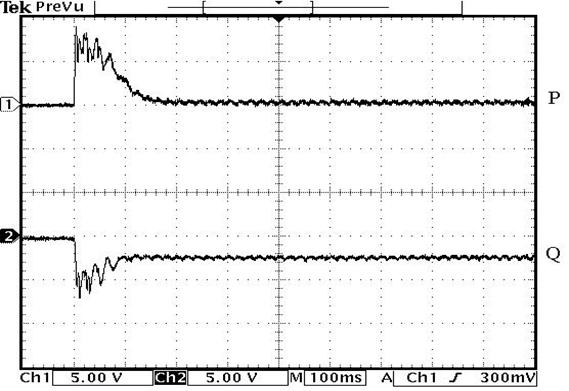Figure 9. Active and reactive power absorbed by induction motor at transient starting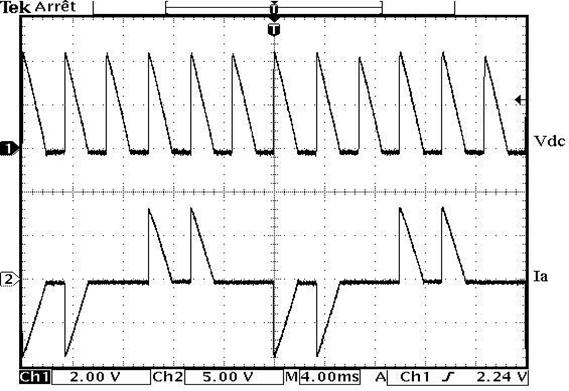Figure 10. Output DC voltage and input current of three phase symmetric rectifier

with α = 80 degree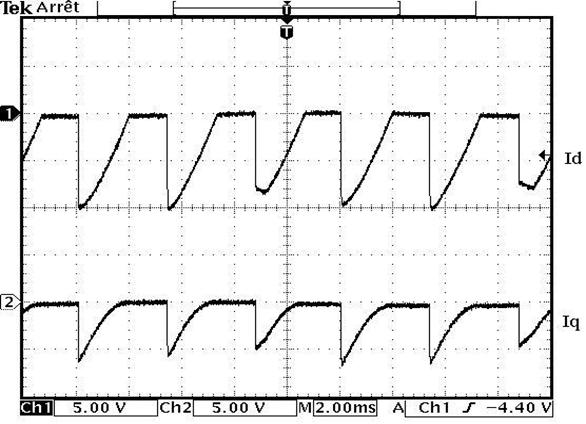Figure 11. Direct and quadrature currents of three phase symmetric rectifier with α = 80°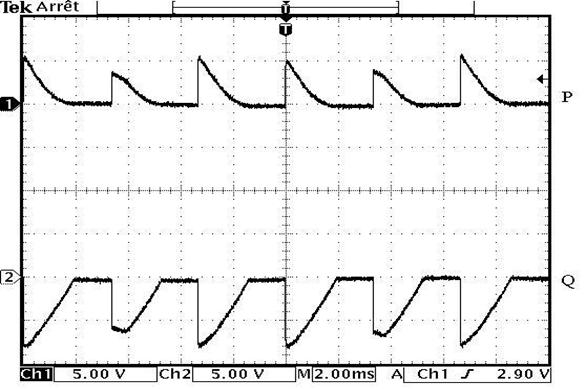Figure 12. Active and reactive power of three phase symmetric rectifier with α = 80 degree

Conclusions

In this paper an electronic measurement of the instantaneous active and reactive power method has been presented. The proposed measurement system has thoroughly been tested in steady and transient states. The measurement method can be used to study different electrical equipment in laboratory as induction and synchronous machines and some converters.

The steady state and transient measurement results obtained have confirmed the applicability of the proposed scheme to design a simple and fast controller for active and reactive power applications.

References

1.      Miller T. J. E., Reactive Power Control in Electric Systems, John Wiley and Sons, Inc. 1982.

2.      Paap C. G., Symmetrical Components in the Time Domain and Their Application to Power Network Calculations, IEEE Trans. On Power Systems, Vol. 15, No. 2, May 2000.

3.      Boisdon C., Drown G., Les systèmes de compensation statique rapide dans les réseaux industriels, R.G.E. No. 12, Decembre 1984.

4.      Tahri A., Draou A., Benghanem M., Power Stability Improvement of Power Utility grid using a New Advanced Static Var Compensator, 23rd International Telecommunications Energy Conference, INTELEC 2001, Edinburgh UK, 14-18 October 2001.

5.      Tahri A., Draou M., Benghanem A., Fast Current Control Strategy of a PWM Inverter used for Static Var Compensation, Proceedings of the 24th Annual Conference of the IEEE Industrial Electronics Society IECON'98, Vol. 1, p. 450-455, Aachen - Germany, August 31 - September 4, 1998.